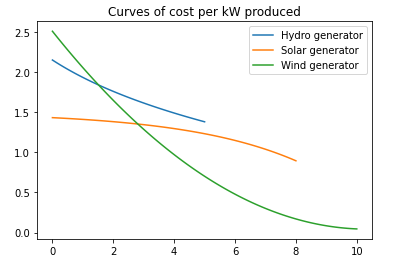top of page
Search

# Power generation Grid Optimization to Optimize the Production of Green Electrical Power

### Power generation grid optimization

We want to optimize the production of green electrical power from 3 kinds of power generators (hydro-based, solar-based and wind-based). The maximum power production of solar and wind generators depends on their primary energy source, on the contrary to hydro generators which have constant maximum power of 5kW per generator. We have predictions for available solar and wind power for the next day:

```# Default imports to work with scipy and matplotlib
import numpy as np
from scipy.optimize import minimize
import matplotlib.pyplot as plt
%matplotlib inline```

```# Global parameters
n = 25   # number of hours (from 0 to n both included)

# Input generators data
hours = np.linspace(0,n-1,n) # 1 data point per hour starting from 0 to n, both included
wind = np.array([7.5,8.3,9.2,8.6,8.63,6.6,7.23,5.21,6.9,4.4,4.3,5.5,5.11,4.33,8.56,8.62,8.43,6.2,6.6,5.81,9.89,8.7,8.8,8.11,8.56]) # in kW
solar = np.array([0,0,0,0,0,0,0.66,2.12,4.23,5.24,6.11,7.09,7.5,8.01,7.98,7.26,6.67,4.12,3.24,2.78,1.27,0,0,0,0])
hydro = np.array([5 for i in range(n)])

# Input data plot
plt.figure()
plt.plot(hours,wind,label="Wind power")
plt.plot(hours,solar,label="Solar power")
plt.plot(hours,hydro,label="Hydro power")
plt.title("Available power (in kW) per generator for next 24 hours")
plt.legend()
plt.show()```

output:Our current power generation grid is based on 12 hydro generators, 10 solar generators and 8 wind generators.

Each kind of generator has specific costs (in euros) and operational constraints (in kW) when used. Some simplified assumptions were done for cost to obtain the following characteristics:In fact, we want to define, per hour, which of the available generators we want to use.

We have to satisfy the following demand:

```n_h = 12 # Number of hydro generators
n_s = 10 # Number of solar generators
n_w = 8  # Number of wind generators

demand = np.array([78.45,80.23,83.34,84.45,85.01,86.98,92.78,93.01,98.23,100.34,106.48,113.52,115.02,111.21,116.82,119.89,118.38,116.63,109.04,102.94,100.02,106.29,102.45,95.93,91.26])

plt.figure()
plt.plot(hours,demand,label='demand')
plt.plot(hours,wind*n_w+solar*n_s+hydro*n_h,label='available')
plt.title("Power demand and total available power (in kW) for next 24 hours")
plt.legend()
plt.show()```

output:### Optimization of current grid

We want to minimize the production cost for the demand of next 24 hours.

1. Define the mathematical optimization problem for only deciding the next hour of production

```import pandas as pd
import numpy as np
from sklearn import linear_model
from sklearn.metrics import mean_absolute_error

x = np.array([hours,wind,hydro,solar]).T
y = np.array(demand)

regr = linear_model.LinearRegression()
regr.fit(x,y)

p = regr.predict(x)

# for next hour

def Average(lst):
return sum(lst) / len(lst)

next_hour_production = regr.predict([[25,Average(wind),Average(hydro),Average(solar)]])

print('next_hour_production : ',next_hour_production)

```

output:

next_hour_production : [110.82051421]

### 2. Solve it for the first hour and validate your model

```#for first hour
hour1 = regr.predict([x])
print('for first hour :', hour1)

print('validatation')
print('absolut error : ',mean_absolute_error(p,y))```

output:

for first hour : 81.10058046908524 validatation absolut error : 3.012548841509367

3. Define and solve the problem over the 24 hours step by step

```plt.figure()
plt.plot(hours,demand,label='demand')
plt.plot(hours,p,label='available')
plt.title("Power demand and total available power (in kW) for next 24 hours")
plt.legend()
plt.show()
x.shape,y.shape```

output:((25, 3), (25,))

Previous assumptions are too basic to be realistics. In fact, the production costs are not linearly dependents on the power production. Then we consider the following functions to compute the cost from the produced power:

• hydro: 𝑐𝑜𝑠𝑡ℎ(𝑝𝑜𝑤𝑒𝑟)=0.88∗𝑙𝑜𝑔(1.18+𝑝𝑜𝑤𝑒𝑟)costh(power)=0.88∗log(1.18+power)

• solar: 𝑐𝑜𝑠𝑡𝑠(𝑝𝑜𝑤𝑒𝑟)=0.05∗𝑒𝑥𝑝(0.47∗𝑝𝑜𝑤𝑒𝑟)costs(power)=0.05∗exp(0.47∗power)

• wind: 𝑐𝑜𝑠𝑡𝑤(𝑝𝑜𝑤𝑒𝑟)=0.0012∗𝑝𝑜𝑤𝑒𝑟3+0.042

```from numpy import log,exp

cost_h=lambda x: 3.27-0.88*log(3.58+x)
cost_s=lambda x: 1.5-0.07*exp(0.27*x)
cost_w=lambda x: 0.023*(x-10.35)**2+0.042

p_h = np.linspace(0,5,100)
p_s = np.linspace(0,8,100)
p_w = np.linspace(0,10,100)
c_h = cost_h(p_h)
c_s = cost_s(p_s)
c_w = cost_w(p_w)

plt.figure()
plt.plot(p_h,c_h,label="Hydro generator")
plt.plot(p_s,c_s,label="Solar generator")
plt.plot(p_w,c_w,label="Wind generator")
plt.title("Curves of cost per kW produced")
plt.legend()
plt.show()```

output:Any query then you can contact us or have a new project related to machine learning or data science then share your requirement details at: